# A Practical Guide for Data Cleaning: Obesity Rate Dataset | How to clean and reformat the raw dataset

The fuel of each and every machine learning or deep learning model is data. Without data, the models are useless. Before building a model and train it, we should try to explore and understand the data at hand. By understanding, I mean correlations, structures, distributions, characteristics and trends in data. A comprehensive understanding of data will be very useful in building a robust and well-designed model. We can draw valuable conclusions by exploring the data. Before we start exploring the data, we need to clean and reformat the dataset so that it can be easily analyzed.

In this post, we will walk through the data cleaning process of “obesity among adults by country” dataset which is available on Kaggle. I will cover the exploratory data analysis part in a separate post. It will be too long to focus for readers if we combine them in a single post.

The dataset contains adult obesity rates in 195 countries between 1975 and 2016. Let’s start by reading the dataset into a Pandas dataframe and take a look at it:]

```import numpy as np
import pandas as pd
df.shape
(198, 127)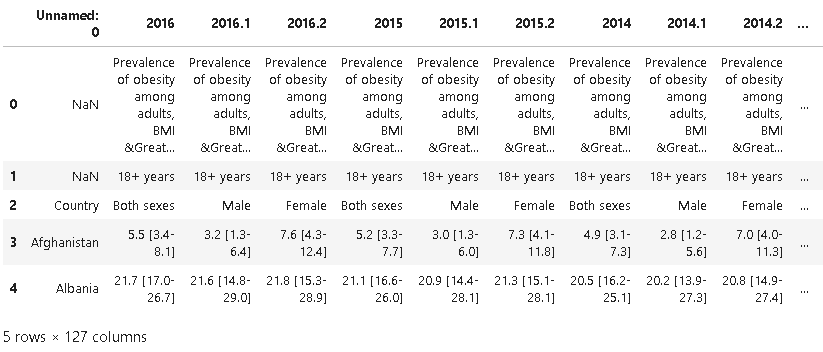It is definitely not in a good-looking format. The first three rows seem like repeating the same information. We can confirm using nunique function on rows:

```print(df.iloc[0, :].unique())
[nan, 'Prevalence of obesity among adults, BMI &GreaterEqual; 30 (age-standardized estimate) (%)']```The first two rows contain the same value in all columns except for the first column and that value is NaN. The third column indicates the gender but we can get the same information from the column names. 2016.1 is male, 2016.2 is female and 2016 is the average and this is valid for all years. The first three rows are redundant so we just drop them.
```df.drop([0,1,2], axis=0, inplace=True)
df.reset_index(drop=True, inplace=True)```

Now the dataframe looks like: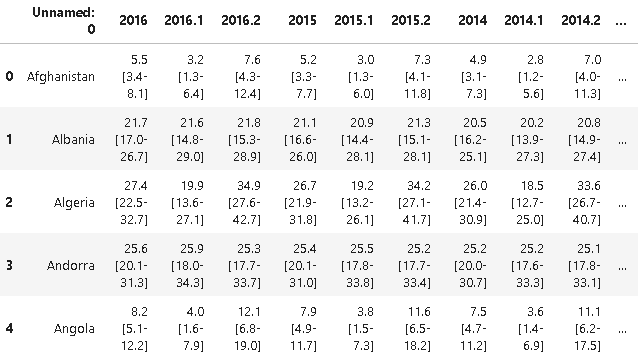The values in plain years (e.g. 2016) are the average of the other two columns of the same year (e.g. 2016.1 and 2016.2). There is no point to keep a column that we can achieve by a simple math operation. So we drop the columns of plain years.

There is a pattern in the column names. Starting from the second column, every third column is a plain year. We can use this pattern to filter column names and pass it to drop function:

```df.drop(df.columns[1::3], axis=1, inplace=True)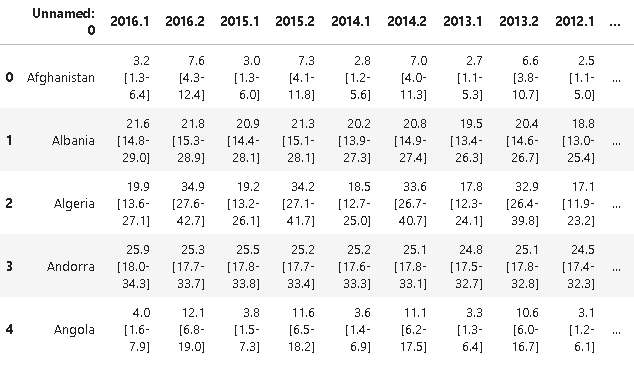The year columns contain a value and a range. The value is the average of upper and lower limit of the range. Thus, we can only take the value as the average obesity rate. We can achieve this by splitting the values and the range, then take the first element after splitting.

```for i in range(1,85):
df.iloc[:,i] = df.iloc[:,i].str.split(expand=True)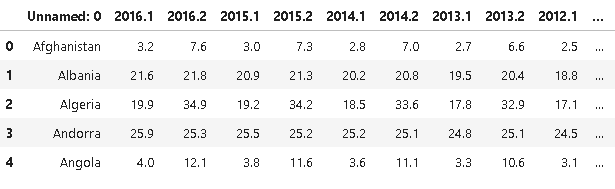This format is not very optimal for analysis. It is better if we have the following columns:
• country, year, gender, obesity_rate

So we need to convert a wide dataframe into a narrow one. Pandas transpose function can be used for this task but there is a much better option which is melt function of pandas. I consider melt function as “smart transpose”.

```df2 = df.melt(id_vars=['Unnamed: 0'], value_name='obesity_rate')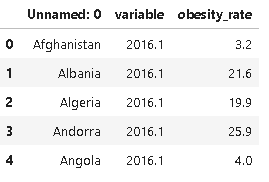We are getting closer to the desired format. The number attached to year indicates the gender. 1 is for male and 2 is for female. We can split variable column into “year” and “gender” columns:

```df2[['year','gender']] = df2.iloc[:,1].str.split('.', expand=True)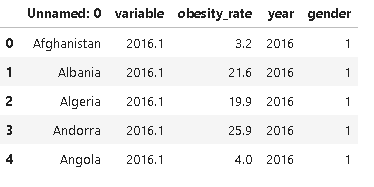There are a few adjustment we need to make:
• Drop the “variable” column because it is represented by “year” and “gender” columns.
• Change the values in “gender” column so that 1 will be male and 2 will be female.
• Change the name of the first columns to “country”
```df2.drop('variable', axis=1, inplace=True)
gender = {'1':'male', '2':'female'}
df2.gender.replace(gender, inplace=True)
df2.rename(columns={'Unnamed: 0':'country'}, inplace=True)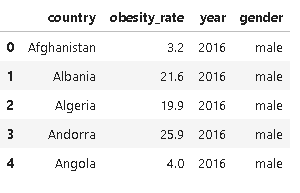Now we have a nice and clean dataframe. Finally, let’s check the shape and datatypes of the new dataframe and also look for missing values.

```df2.shape
(16380, 4)
df2.isna().sum()

country         0
obesity_rate    0
year            0
gender          0
dtype: int64
df2.dtypes
country         object
obesity_rate    object
year            object
gender          object
dtype: object```

We need to change the datatype of “obesity_rate” column to a float. When I tried to convert the values, I found out that there are “No” values in obesity_rate column which cannot be converted to a numerical value. After checking the “No” values, I saw that only a few countries include “No” values in obesity_rate column:

```df2[df2.obesity_rate == 'No']['country'].unique()
array(['Monaco', 'San Marino', 'South Sudan', 'Sudan'], dtype=object)```

Actually, all the values of these countries are “No”:

```omit = df2[df2.obesity_rate == 'No']['country'].unique()
df2[df2.country.isin(omit)]['obesity_rate'].unique()
df2 = df2[~df2.country.isin(omit)]```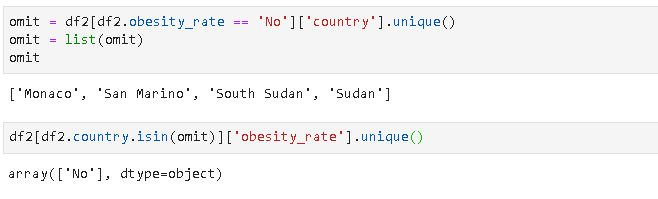So we just drop them:

`df2 = df2[~df2.country.isin(omit)]`
Tilde (~) operator means NOT so we take the rows that the country is not in the omit list. We can now change the datatype to numeric:

```df2 = df2.astype({'obesity_rate': 'float32'})
df2.dtypes```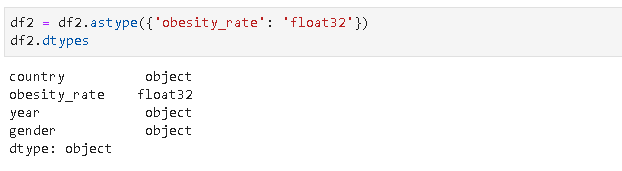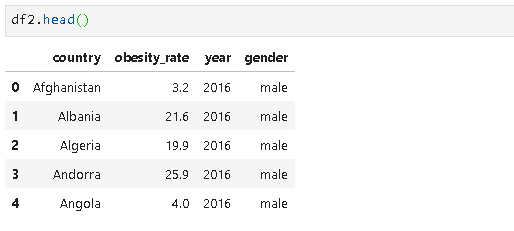We now have a clean dataframe in an appropriate format for exploratory data analysis (EDA). I will cover a detailed EDA process with informative visualizations in the next post.

“A Practical Guide for Data Cleaning: Obesity Rate Dataset | How to clean and reformat the raw dataset”
– Soner Yıldırım

### Related Stories

Nov 25, 2021

#### 5 Tips To Ace Your Job Interview For A Data Scientist Opening

5 Tips To Ace Your Job Interview For A Data Scientist Opening.PNG 795.94 KBImage SourceAspiring data scientists have a bright future ahead of them....By Daniel Morales
Nov 12, 2021

#### When to Avoid Deep LearningBy Matt Przybyla
Oct 16, 2021

#### 6 Advanced Statistical Concepts in Data Science

The article contains some of the most commonly used advanced statistical concepts along with their Python implementation.In my previous articles Be...By Nagesh Singh Chauhan